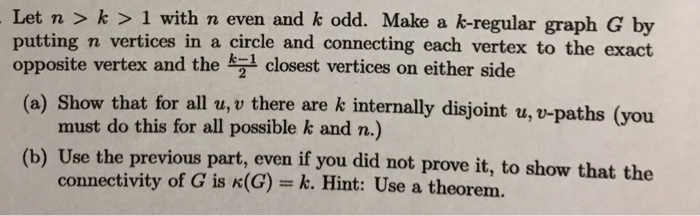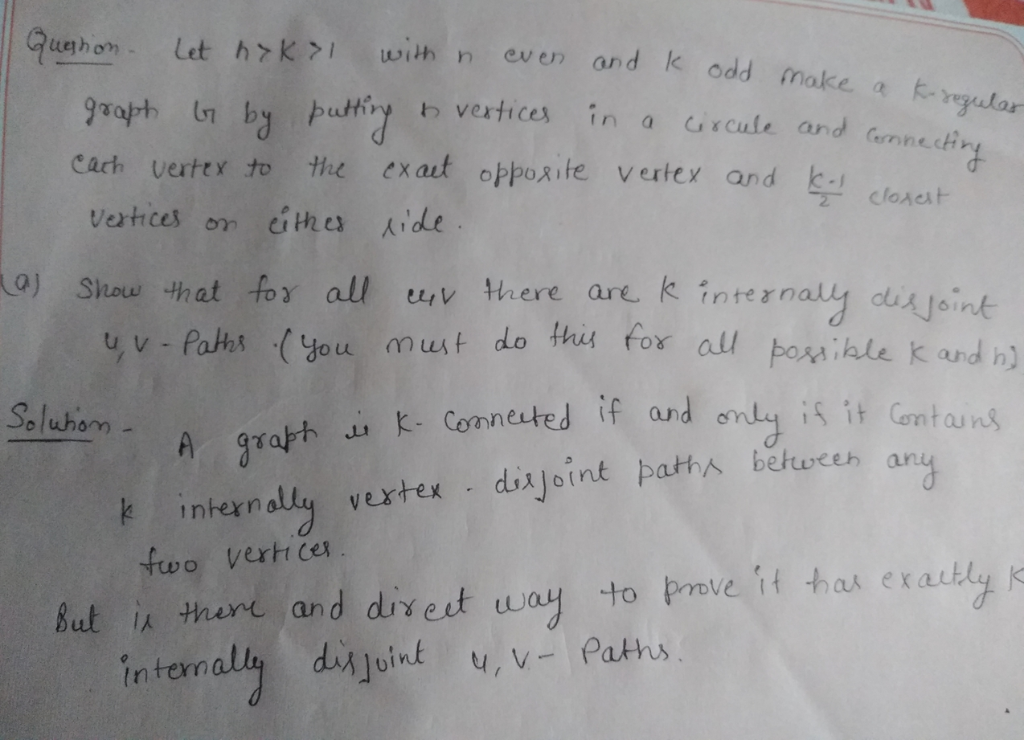Homework Help Question & Answers

Let n >k> 1 with n even and k odd. Make a k-regular graph G by putting n vertices in a circle and...

need help with a and b in this graph theory questionLet n >k> 1 with n even and k odd. Make a k-regular graph G by putting n vertices in a circle and connecting each vertex to the exact a) Show that for all u,v there are k internally disjoint u, v-paths (you (b) Use the previous part, even if you did not prove it, to show that the e vertex and the k 1 closest vertices on either side must do this for all possible k and n.) connectivity of G is k(G) k. Hint: Use a theoremAdd Answer of: Let n >k> 1 with n even and k odd. Make a k-regular graph G by putting n vertices in a circle and...
More Homework Help Questions Additional questions in this topic.

• Recall the definition of the degree of a vertex in a graph. a) Suppose a graph has 7 vertices, each of degree 2 or 3. Is the graph necessarily connected ? b) Now the graph has 7 vertices, each degree...

Need Online Homework Help?

Get FREE EXPERT Answers
WITHIN MINUTES
Related Questions